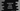# JavaScript array some() function explanation with examples## JavaScript array some function:

The some function of JavaScript array is used to check if at least one element in an array passed for a given function or not. It takes a callback function as the parameter and uses that function with every element in the array. If at least one element passes the test implemented by this function, it will return true. Else, if it fails for all elements in the array, it will return false.

In this post, we will learn how to use this function with different examples.

### Definition of some():

some() is defined as like below:

#### some() with arrow functions:

``````some((e) => {...})
some((e, i) => {...})
some((e, i, arr) => {...})``````

Here, e is the current element it is iterating, i is the index of this element and arr is the array where some is called. Both i and arr are optional values.

#### some() with callback function:

We can also pass a callback function to some() as the parameter. It will be as like below:

``some(f, thisArg)``

Where, f is the callback function and thisArg is the value to use as this when executing f. This is an optional value.

#### some() with inline callback function:

We can also use it with inline callback function. It looks like as below:

``````some(function(e) { })
some(function(e, i) { })
some(function(e, i, arr){  })
some(function(e, i, arr) { }, thisArg)``````

Here, we are using an inline callback function. Where,

• e is the current element it is iterating.
• i is the index of the current element being processed. It is an optional value.
• arr is the array. This is an optional value.
• thisArg is to use as this while using the callback function.

### Return value of some:

This method returns a boolean value. It returns true if the callback function returns true for at least one value in the array. Otherwise it will return false.

This method executes the callback function for each element of the array one by one. If it gets true for any element, it returns true immediately. If it finds false for all of the elements in the array, it returns false.

### Example of some():

Let’s try some() in different way:

### some() with arrow function:

Let’s try some with arrow functions. We will use an arrow function to check if some elements of an array are even or not.

``````let firstArray = [2, 3, 4, 5, 6, 7, 8];
let secondArray = [1, 3, 5, 7, 9, 11];

console.log(firstArray.some(e => e%2 === 0));
console.log(secondArray.some(e => e%2 === 0));``````

In this example, we are checking if some numbers are even or not. We have two arrays, firstArray and secondArray. We are using an arrow function to check if some of the numbers in the arrays are even or not.

If you run this program, it will print:

``````true
false``````

As you can see here, for the firstArray, it returns true because there are even numbers in this array. Similarly, for the secondArray, there are no even numbers and so it returns false.

### some() with callback function:

Let’s write down the same example with a callback function. We can write a separate callback function and use it in the some function.

``````function isSomeEven(e, i, arr) {
return e % 2 === 0;
}

let firstArray = [2, 3, 4, 5, 6, 7, 8];
let secondArray = [1, 3, 5, 7, 9, 11];

console.log(firstArray.some(isSomeEven));
console.log(secondArray.some(isSomeEven));``````

We created a new function isSomeEven and passed this to some method. It will result similar output.

### some() with inline callback function:

Let’s try some with inline callback:

``````let firstArray = [2, 3, 4, 5, 6, 7, 8];
let secondArray = [1, 3, 5, 7, 9, 11];

console.log(firstArray.some(function isSomeEven(e, i, arr) {
return e % 2 === 0;
}));
console.log(secondArray.some(function isSomeEven(e, i, arr) {
return e % 2 === 0;
}));``````

It will give the same output.